Related Articles

# Mahotas – Element Structure for Dilating Image

• Last Updated : 29 Jul, 2021

In this article we will see how we can set the element structure of the dilate of image in mahotas. Dilation adds pixels to the boundaries of objects in an image, while erosion removes pixels on object boundaries. The number of pixels added or removed from the objects in an image depends on the size and shape of the structuring element used to process the image. In order to dilate the image we use mahotas.morph.dilate method.By setting element structure we can increase or decrease the dilating effect on the image.

In this tutorial we will use “luispedro” image, below is the command to load it.

`mahotas.demos.load('luispedro')`

Below is the luispedro image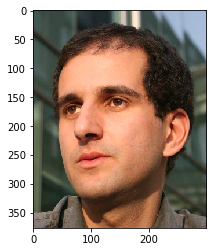Implementation steps :
2. Filter the image
3. Use otsu method for threshold of the image
4. Create a structure of the element with the help of numpy ndarray for binary values
5. Use the element for dilating the image

Below is the implementation

## Python3

 `# importing required libraries``import` `mahotas``import` `mahotas.demos``from` `pylab ``import` `gray, imshow, show``import` `numpy as np`` ` `# loading image``luispedro ``=` `mahotas.demos.load(``'luispedro'``)`` ` `# filtering image``luispedro ``=` `luispedro.``max``(``2``)`` ` `# otsu method``T_otsu ``=` `mahotas.otsu(luispedro)``  ` `# image values should be greater than otsu value``img ``=` `luispedro > T_otsu`` ` `print``(``"Image threshold using Otsu Method"``)`` ` `# showing image``imshow(img)``show()`` ` `# erode structure``es ``=` `np.array([``        ``[``1``, ``1``, ``1``, ``1``],``        ``[``1``, ``1``, ``1``, ``1``],``        ``[``1``, ``1``, ``1``, ``1``],``        ``[``1``, ``1``, ``1``, ``1``]], ``bool``)` `# dilating image``dilate_img ``=` `mahotas.morph.dilate(img, es)`` ` `# showing dilated image``print``(``"Dilated Image"``)``imshow(dilate_img)``show()`

Output :

`Image threshold using Otsu Method`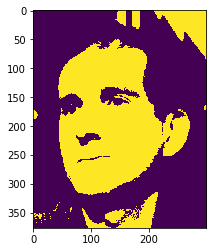`Dilated Image`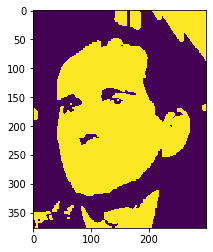Another example

## Python3

 `# importing required libraries``import` `mahotas``import` `numpy as np``import` `matplotlib.pyplot as plt``import` `os`` ` `# loading image``img ``=` `mahotas.imread(``'dog_image.png'``)``      ` `# setting filter to the image``img ``=` `img[:, :, ``0``]` `# otsu method``T_otsu ``=` `mahotas.otsu(img)`` `  `# image values should be greater than otsu value``img ``=` `img > T_otsu` `print``(``"Image threshold using Otsu Method"``)` `# showing image``imshow(img)``show()` `# erode structure``es ``=` `np.array([``        ``[``1``, ``1``, ``1``, ``1``],``        ``[``1``, ``1``, ``1``, ``1``],``        ``[``1``, ``1``, ``1``, ``1``],``        ``[``1``, ``1``, ``1``, ``1``]], ``bool``)` `# dilating image``dilate_img ``=` `mahotas.morph.dilate(img, es)`` ` `# showing dilated image``print``(``"Dilated Image"``)``imshow(dilate_img)``show()`

Output :

`Image threshold using Otsu Method`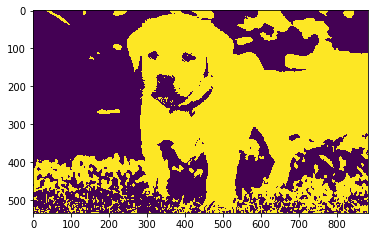`Dilated Image`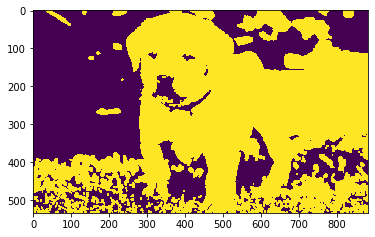Attention geek! Strengthen your foundations with the Python Programming Foundation Course and learn the basics.

To begin with, your interview preparations Enhance your Data Structures concepts with the Python DS Course. And to begin with your Machine Learning Journey, join the Machine Learning – Basic Level Course

My Personal Notes arrow_drop_up